Open in App
Not now

# Perl | Creating Excel Files

• Last Updated : 07 May, 2019

Excel files are the most commonly used office application to communicate between computers. It is used to create rows and columns of text, numbers, and formulas for calculations. It is a good way to send reports. This demonstration works on Linux, Windows and other platforms as well. In excel, rows are numbered from 1 to n… and columns are marked by letters from A, B, C and so on.. hence, A1 refers to the top left corner. For creating excel files with Perl you can use padre IDE, we will also use Excel::Writer::XLSX module.
Perl uses write() function to add content to the excel file.

Parameters:
content: which is to be added to the worksheet.

Creating an Excel File

Excel Files can be created using Perl command line but first we need to load Excel::Writer::XLSX module.

 `#!/usr/bin/perl  ` `use` `Excel::Writer::XLSX; ` ` `  `my` `\$Excelbook` `= Excel::Writer::XLSX->new( ``'GFG_Sample.xlsx'` `); ` `my` `\$Excelsheet` `= ``\$Excelbook``->add_worksheet(); ` ` `  `\$Excelsheet``->``write``( ``"A1"``, ``"Hello!"` `); ` `\$Excelsheet``->``write``( ``"A2"``, ``"GeeksForGeeks"` `); ` `\$Excelsheet``->``write``( ``"B1"``, ``"Next_Column"` `); ` ` `  `\$Excelbook``->``close``; `

Output: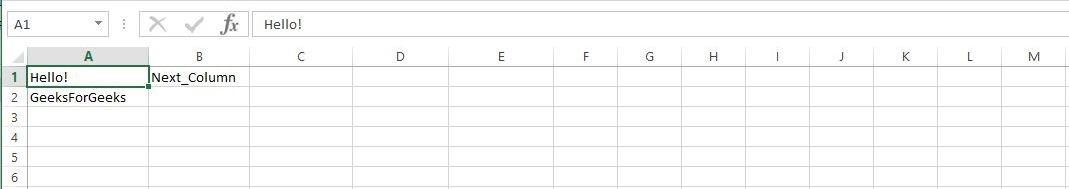Here is how the Program works:
Step 1: Load the module Excel::Writer::XLSX.
Step 2: Create an object \$Excelbook which represents the whole Excel File.
Step 3: Call write() method to add data to the worksheet.
Step 4: Now, save the file with .pl extension.
Step 5: Run your .pl file on command line and Excelsheet will be created.

Use of Basic Formulas

Excel provides the use of various Mathematical Formulae for the ease of calculations on the excelsheets like balance sheet, business records, etc.
Here is the description of two basic formulas of Excel:

Excel provides a method ‘SUM’ for the addition of values on specific cells.

Syntax: =SUM(Start, End)

Parameter:
Start: Address of the starting cell
End: Address of the Ending cell

Returns: the summation of values between the Starting and Ending cell.

 `#!/usr/bin/perl  ` `use` `Excel::Writer::XLSX; ` ` `  `my` `\$Excelbook` `= Excel::Writer::XLSX->new( ``'GFG_Sample.xlsx'` `); ` `my` `\$Excelsheet` `= ``\$Excelbook``->add_worksheet(); ` ` `  `# Writing values at A1 and A2 ` `\$Excelsheet``->``write``( ``"A1"``, 55 ); ` `\$Excelsheet``->``write``( ``"A2"``, 47 ); ` ` `  `# Adding without use of SUM method ` `\$Excelsheet``->``write``( ``"A3"``, ``"= A1 + A2"` `); ` ` `  `# Addition of a Range of cells ` `\$Excelsheet``->``write``( ``"A4"``, ``" =SUM(A1:A3)"` `); `

Output: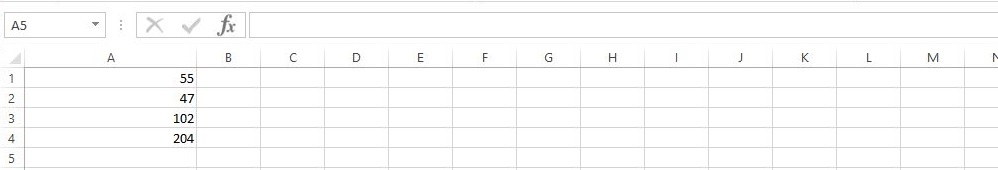• Count:
This function in Excel is used to count all the cells in the given range which contain only numeric value.

Syntax: =COUNT(Start, End)
Returns: count of all cells containing numeric value

 `#!/usr/bin/perl  ` `use` `Excel::Writer::XLSX; ` ` `  `my` `\$Excelbook` `= Excel::Writer::XLSX->new( ``'GFG_Sample.xlsx'` `); ` `my` `\$Excelsheet` `= ``\$Excelbook``->add_worksheet(); ` ` `  `# Writing values ` `\$Excelsheet``->``write``( ``"A1"``, 5 ); ` `\$Excelsheet``->``write``( ``"A2"``, 40 ); ` `\$Excelsheet``->``write``( ``"A3"``, ``"Hello"` `); ` `\$Excelsheet``->``write``( ``"A4"``, 10 ); ` ` `  `# Addition of a Range of cells ` `\$Excelsheet``->``write``( ``"A5"``, ``"Count ="``); ` `\$Excelsheet``->``write``( ``"B5"``, ``"=COUNT(A1:A4)"` `); `

Output: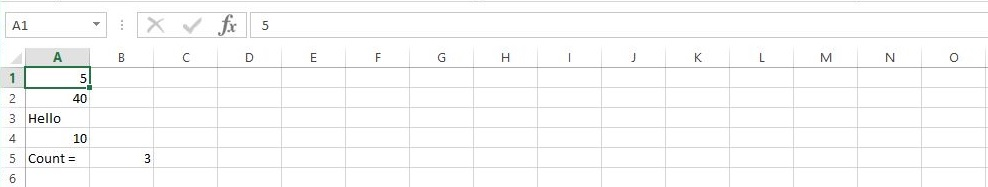Colors can be used in ExcelSheets to mark specific values separately. These colors are specified with the use of add_format() method.

 `#!/usr/bin/perl ` `use` `Excel::Writer::XLSX; ` ` `  `my` `\$Excelbook` `= Excel::Writer::XLSX->new( ``'GFG_Sample.xlsx'` `); ` `my` `\$Excelsheet` `= ``\$Excelbook``->add_worksheet(); ` ` `  `# Setting value of color ` `my` `\$color1` `= ``\$Excelbook``->add_format(``color``=> ``'blue'``,); ` `my` `\$color2` `= ``\$Excelbook``->add_format(``color``=> ``'red'``,); ` `my` `\$color3` `= ``\$Excelbook``->add_format(``color``=> ``'green'``,); ` ` `  `\$Excelsheet``->``write``( ``"A2"``, ``"Geeks"``, ``\$color1` `); ` `\$Excelsheet``->``write``( ``"B2"``, ``"For"``, ``\$color2` `); ` `\$Excelsheet``->``write``( ``"C2"``, ``"Geeks"``, ``\$color3` `); ` `\$Excelbook``->``close``; `

Output: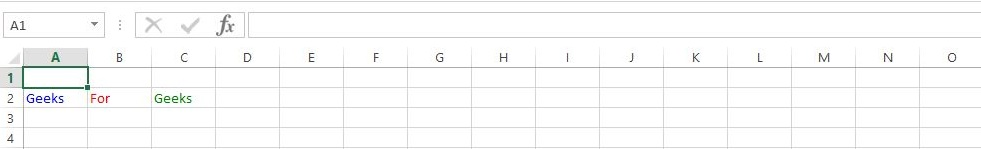Values can be added at specific coordinates by providing address of cells at which value is to be added.

Syntax: write(R,C, “value”)
Parameters:
R and C are the coordinates of the Row and Column respectively.

 `#!/usr/bin/perl  ` `use` `Excel::Writer::XLSX; ` ` `  `my` `\$Excelbook` `= Excel::Writer::XLSX->new( ``'GFG_Sample.xlsx'` `); ` `my` `\$Excelsheet` `= ``\$Excelbook``->add_worksheet(); ` ` `  `\$Excelsheet``->``write``( 0, 0, ``"Hello!"` `); ` `\$Excelsheet``->``write``( 1, 0, ``"GeeksForGeeks"` `); ` `\$Excelsheet``->``write``( 3, 2, ``"Welcome!!!"` `); ` ` `  `\$Excelbook``->``close``; `

Output: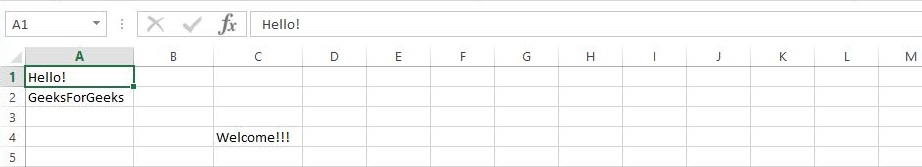My Personal Notes arrow_drop_up
Related Articles